## New versions of Maxima and Scilab released

December 20th, 2009

It’s been a great week for Open Source maths software this week with new releases of both Maxima and Scilab.

Maxima is a computer algebra system written in lisp that runs on most operating systems including Linux, Mac OS X and Windows.  The latest version, Maxima 5.20.1,  was released on Sunday 13th December and the full list of changes can be found here.  Highlights include improvements to the calculation of special functions, faster fourier transform routines and a general mechanism for functions to distribute over operators.

This last item is pretty cool since it allows you to map functions over lists in a similar manner to Mathematica.  For example, if you apply the sin function to a list in Maxima then you’ll now get a list containing the sines of each element of that list:

(%i1) sin([1,2,3]);
(%o1) [sin(1),sin(2),sin(3)]

Here are some examples of this new functionality for two-argument functions such as expintegral_e (taken from a post of Dieter Kaiser’s) include :

(%i2) expintegral_e([1,2,3],x);
(%o2) [expintegral_e(1,x),expintegral_e(2,x),expintegral_e(3,x)]

(%i3) expintegral_e(1,[x,y,z]);
(%o3) [expintegral_e(1,x),expintegral_e(1,y),expintegral_e(1,z)]

(%i4) expintegral_e([1,2,3],[x,y,z]);
(%o4) [[expintegral_e(1,x),expintegral_e(1,y),expintegral_e(1,z)],
[expintegral_e(2,x),expintegral_e(2,y),expintegral_e(2,z)],
[expintegral_e(3,x),expintegral_e(3,y),expintegral_e(3,z)]]

Scilab is a fantastic open source numerical mathematics environment that always seems to come top of the list when people start discussing free MATLAB alternatives.  December 17th saw the release of Scilab 5.20 and the list of changes is epic (warning:pdf file).

The first new Scilab feature that caught my eye is the addition of a module called Xcos.  Xcos 1.0 is based on Scicos 4.3 which is a “graphical dynamical system modeler and simulator.”  Now I’ve not used any of this stuff before but as far as I can tell it could be considered as a free version of Simulink.  A bit of googling turned up a paper by M.G.J.M. Maassen, a student at the Eindoven University of Technology, who looked at the issue of migrating from Simulink to Scicos with respect to real time programs.  Written in 2006, this paper is slightly out of date but is a great start for anyone who is considering moving away from Simulink.

Along with other free mathematical software applications such as Octave, Sage, Python/Numpy and freemat, these new releases demonstrate that the world of free, open source mathematical applications has never looked better.

## The rise and rise of Python in computational science

December 16th, 2009

One of my plans for next year is to start giving short talks and tutorials about scientific Python to various groups around the University of Manchester.  Ever since I attended EuroSciPy in Leipzig earlier this year I have been excited about the possibilities offered by Python for students, researchers and teachers.  I genuinely believe that Python and SAGE will do for MATLAB and Mathematica users what R has done for users of SPSS and Stata.  It’s the future…I’ve tasted it!  Here are a few links to others who agree with me.

Earlier this week I mentioned my plans to a colleague of mine over coffee and he said “Cool, I didn’t realise that you were a Python expert!”

Of course I’m NOT an expert on Python but it turns out that you just don’t have to be an expert in Python in order to get useful stuff done and THAT is my point!

## Using SAGE to investigate the discrete logistic equation

December 10th, 2009

There have been a couple of blog posts recently that have focused on creating interactive demonstrations for the discrete logistic equation – a highly simplified model of population growth that is often used as an example of how chaotic solutions can arise in simple systems.  The first blog post over at Division by Zero used the free GeoGebra package to create the demonstration and a follow up post over at MathRecreation used a proprietary package called Fathom (which I have to confess I have never heard of – drop me a line if you have used it and like it).  Finally, there is also a Mathematica demonstration of the discrete logistic equation over at the Wolfram Demonstrations project.

I figured that one more demonstration wouldn’t hurt so I coded it up in SAGE – a free open source mathematical package that has a level of power on par with Mathematica or MATLAB.  Here’s the code (click here if you’d prefer to download it as a file).

def newpop(m,prevpop):
return m*prevpop*(1-prevpop)

def populationhistory(startpop,m,length):
history = [startpop]
for i in range(length):
history.append( newpop(m,history[i]) )
return history

@interact
def _( m=slider(0.05,5,0.05,default=1.75,label='Malthus Factor') ):
myplot=list_plot( populationhistory(0.1,m,20) ,plotjoined=True,marker='o',ymin=0,ymax=1)
myplot.show()

Here’s a screenshot for a Malthus Factor of 1.75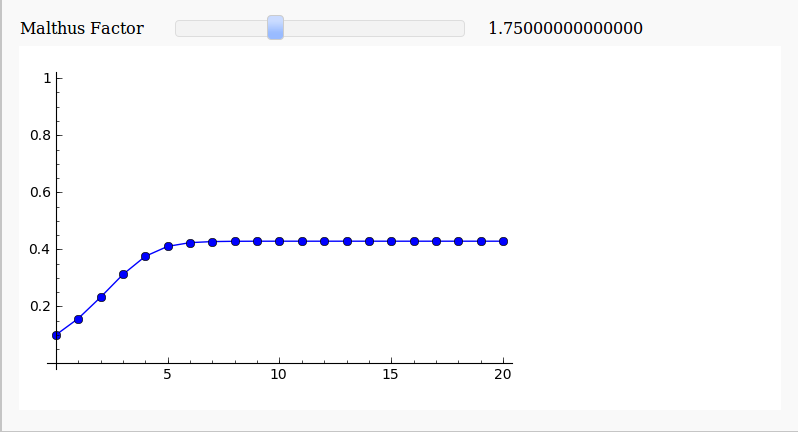and here’s one for a Malthus Factor of 3.1There are now so many different ways to easily make interactive mathematical demonstrations that there really is no excuse not to use them.

Update (11th December 2009)

As Harald Schilly points out, you can make a nice fractal out of this by plotting the limit points.  My blogging software ruined his code when he placed it in the comments section so I reproduce it here (but he’s also uploaded it to sagenb)

var('x malthus')
step(x,malthus) = malthus * x * (1-x)
stepfast = fast_callable(step, vars=[x, malthus], domain=RDF)

def logistic(m, step):
# filter cycles
v = .5
for i in range(100):
v = stepfast(v,m)
points = []
for i in range(100):
v = stepfast(v,m)
points.append((m+step*random(),v))
return points
points=[]
step = 0.005
for m in sxrange(2.5,4,step):
points += logistic(m, step)
point(points,pointsize=1).show(dpi=150)## Adding a MATLAB icon to the GNOME Applications menu in Ubuntu

November 24th, 2009

One of the problems with the Linux MATLAB installer is that it doesn’t add any items to the menu in windows managers such as GNOME or KDE so you have to do it manually.  Now, there are several ways to do this but one of the easiest is to use the GUI tools provided with Ubuntu (assuming you are using Ubuntu of course).  For the record I am using Ubuntu 9.10 (Karmic Koala) with the default GNOME windows manager along with  MATLAB 2009b but I imagine that these instructions will work for a few other configurations too.

First things first, you’ll want to download a nice, scalable MATLAB icon since the ones included with MATLAB itself are a bit crude to say the least.  I tried to create one directly from MATLAB using the logo command and the Scalable Vector Graphics (SVG) Export of Figures package on The File Exchange but the result wasn’t very good (see below).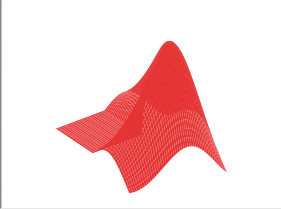Fortunately someone called malte has created a much nicer .svg file (using inkscape) that looks just like the MATLAB logo and made it available via his blog.

Once you have the svg file you can start creating the shortcut as follows:

• On the GNOME Desktop click on System->Preferences->Main Menu
• Once the Main Menu program has started choose where you want your MATLAB icon to go and click on New Item.  In the screenshot below I have started to put it in the Programming submenu.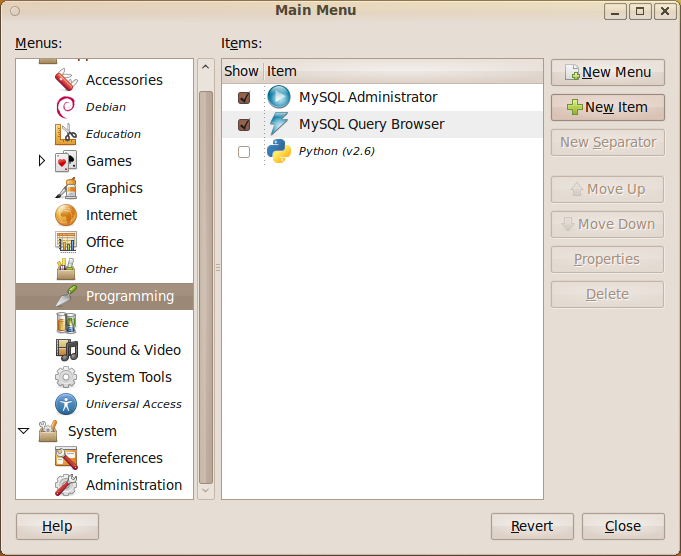• Give your shortcut a name and put matlab -desktop in the command field. If you didn’t create a shortcut to the matlab executable when you installed the program then you may need to put the full path in instead (i.e. something like /opt/MATLAB/2009b/bin/matlab )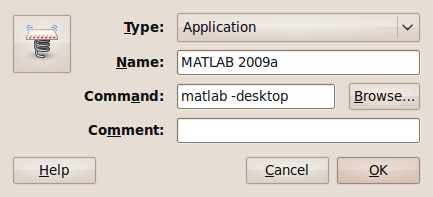• Now let’s sort out the icon. Click on the spring on the left hand side of the above window to get the window below.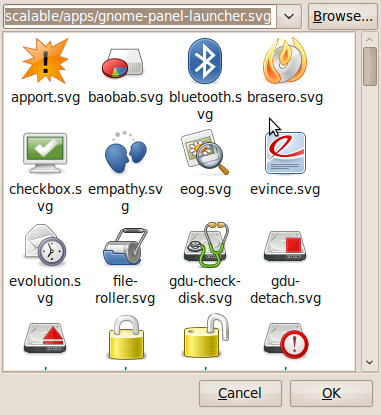• Click on Browse and then select the folder that contains the .svg file you downloaded from malte’s blog.  I put it in my Desktop folder for the screenshot below.  Note that the svg file doesn’t appear in the preview pane.  Click on Open.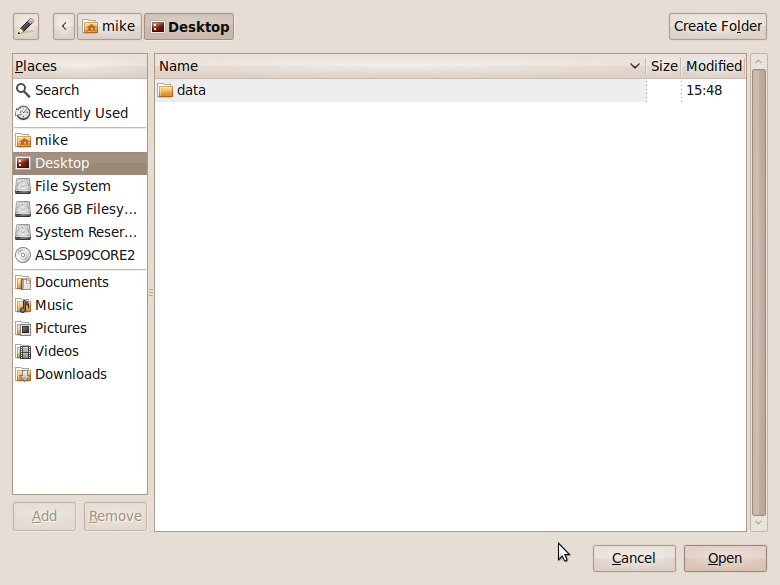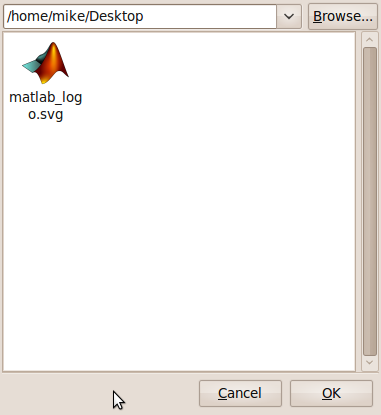• Click on Close and you are done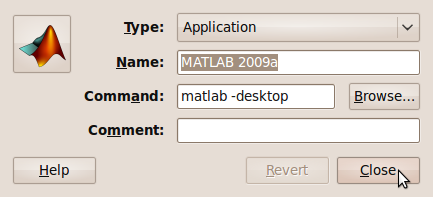If you are using the Student version of MATLAB then the process is slightly different and has been covered by my friend Paul Brabban over at CrossedStreams.com.

## Interactive Fourier Series with SAGE math

November 17th, 2009

Earlier today I was chatting to a lecturer over coffee about various mathematical packages that he might use for an upcoming Masters course  (note – offer me food or drink and I’m happy to talk about pretty much anything). He was mainly interested in Mathematica and so we spent most of our time discussing that but it is part of my job to make sure that he considers all of the alternatives – both commercial and open source. The course he was planning on running (which I’ll keep to myself out of respect for his confidentiality) was definitely a good fit for Mathematica but I felt that SAGE might suite him nicely as well.

“Does it have nice, interactive functionality like Mathematica’s Manipulate function?” he asked

Oh yes! Here is a toy example that I coded up in about the same amount of time that it took to write the introductory paragraph above (but hopefully it has no mistakes). With just a bit of effort pretty much anyone can make fully interactive mathematical demonstrations using completely free software. For more examples of SAGE’s interactive functionality check out their wiki.Here’s the code:

def ftermSquare(n):
return(1/n*sin(n*x*pi/3))

def ftermSawtooth(n):
return(1/n*sin(n*x*pi/3))

def ftermParabola(n):
return((-1)^n/n^2 * cos(n*x))

def fseriesSquare(n):
return(4/pi*sum(ftermSquare(i) for i in range (1,2*n,2)))

def fseriesSawtooth(n):
return(1/2-1/pi*sum(ftermSawtooth(i) for i in range (1,n)))

def fseriesParabola(n):
return(pi^2/3 + 4*sum(ftermParabola(i) for i in range(1,n)))

@interact
def plotFourier(n=slider(1, 30,1,10,'Number of terms')
,plotpoints=('Value of plot_points',[100,500,1000]),Function=['Saw Tooth','Square Wave','Periodic Parabola']):
if Function=='Saw Tooth':
show(plot(fseriesSawtooth(n),x,-6,6,plot_points=plotpoints))
if Function=='Square Wave':
show(plot(fseriesSquare(n),x,-6,6,plot_points=plotpoints))
if Function=='Periodic Parabola':
show(plot(fseriesParabola(n),x,-6,6,plot_points=plotpoints))

## Right click to encrypt files in Ubuntu 9.10

November 3rd, 2009

I’ve just installed Ubuntu version 9.10 (Karmic Koala) from scratch on my new work machine and noticed that you can no longer right click on a file to encrypt it. The functionality is still there – it just isn’t available by default. To get it just install the seahorse-plugins package as follows

sudo apt-get install seahorse-plugins


I did a reboot to get the changes to take but there is probably a less drastic way of getting the job done.

## MATLAB and R ‘Rosetta Stone’

September 21st, 2009

I was recently given the task of converting a small piece of code written in R, the free open-source programming language heavily used by statisticians, into MATLAB which was an interesting exercise since I had never coded a single line of R in my life!  Fortunately for me, the code was rather simple and I didn’t have too much trouble with it but other people may not be so lucky since both MATLAB and R can be rather complicated to say the least.  Wouldn’t it be nice if there was a sort of Rosetta Stone that helped you to translate between the two systems?

Happily, it turns out that there is in the form of The MATLAB / R Reference by David Hiebeler which gives both the R and MATLAB commands for hundreds of common (and some not so common) operations.

While flicking through the 47 page document I noted that there are a few MATLAB commands for which David hasn’t found an R equivalent (possibly because there simply isn’t one of course).  For example, at number 161 of David’s document he describes the MATLAB command

yy=spline(x,y,xx)

which he describes as

‘Fit cubic spline with “not-a-knot” conditions (the first two piecewise cubics coincide,as do the last two), to points (xi , yi ) whose coordinates are in vectors x and y; evaluate at points whose x coordinates are in vector xx, storing corresponding y’s in yy.’

At the moment David doesn’t know of an R equivalent so if you are a R master then maybe you could help out with this extremely useful document?

## SAGE math 3.4.1 released

April 27th, 2009

The latest version of the powerful free, open-source maths package, SAGE, was released last week.  Version 3.4.1 brings us a lot of new functionality compared to 3.4 and the SAGE team have prepared a detailed document showing us why the upgrade is worthwhile.

For example, the new complex_plot function looks fantastic.  From the documentation:

The function complex_plot() takes a complex function f(z) of one variable and plots output of the function over the specified xrange and yrange. The magnitude of the output is indicated by the brightness (with zero being black and infinity being white), while the argument is represented by the hue with red being positive real and increasing through orange, yellow, etc. as the argument increases.

sage: f(z) = z^5 + z - 1 + 1/z
sage: complex_plot(f, (-3, 3), (-3, 3))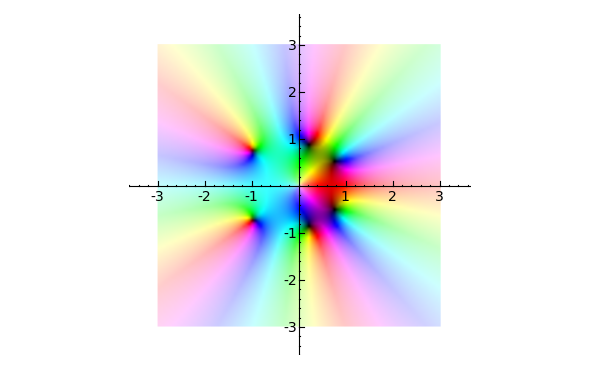Sage aims to become a ‘viable free open source alternative to Magma, Maple, Mathematica and Matlab’ and I think it is well on the way.  I become a little more impressed with it with every release and I am a hardcore Mathematica and MATLAB fan.

One major problem with it (IMHO at least) is that there is no native windows version which prevents a lot of casual users from trying it out.  Although you can get it working on Windows, it is far from ideal because you have to run a virtual machine image using VMWare player.  The hard-core techno geeks among you might well be thinking ‘So what?  Sounds easy enough.’ but it’s an extra level of complexity that casual users simply do not want to have to concern themselves with.  Of course there is also the issue of performance – emulating an entire machine to run a single application is hardly a good use of compute resources.

There is a good reason why SAGE doesn’t have a proper Windows version yet – it’s based upon a lot of component parts that don’t have Windows versions and someone has to port each and every one of them.  It’s going to be a lot of work but I think it will be worth it.

When the SAGE development team release a native Windows version of their software then I have no doubt that it will make a significant impact on the mathematical software scene – especially in education.  There will be nothing preventing every school and university in the world from having access to a world-class computer algebra system.

In an ideal world everyone would be running Linux but we don’t live in an ideal world so a Windows version of SAGE would be a step in the right direction.

Update: It turns out that a Windows port is being developed and something should be ready soon – http://windows.sagemath.org/ Thanks to mvngu in the comments section for pointing this out. I should have done my research better!

## Ocatve 3.0.5 released

April 9th, 2009

A new version of the popular free, open source MATLAB clone, Octave, was released yesterday containing a fix to a bug introduced in the previous version, 3.0.4 which itself was only released last week containing yet more bug fixes.

Octave is a fantastic project and it’s great to see that the development team responds to bugs in the code so quickly.

## R Compared to MATLAB (or ‘learning a thing or two from your students’)

March 23rd, 2009

Near the end of last week I was acting as teaching assistant for a MATLAB course and some of the students wanted to know if there were any viable free, open source alternatives to MATLAB.  I duly listed what I considered to be the standard set – Scilab, Octave, SAGE and Python – which are more or less MATLABy depending on your point of view.

One student asked ‘What about R?’ and I had to confess that I didn’t know much about it except that it is used a lot by statisticians and is essentially a free, open source version of S+.  I ventured the opinion that maybe R was too specialised to be considered a general MATLAB alternative with the caveat that I didn’t actually know what I was talking about.

It seems that there is nothing a student likes more than to teach the teacher and so much googling ensued.  I was pointed to a small set of speed comparisons between MATLAB and R for example which includes some matrix operations like finding eigenvalues and generating Toeplitz matrices.  You don’t get much more MATLABy than matrices!  Other articles such as this comparison between various data analysis packages also proved interesting and useful.

So, thanks to a line of questioning from some MATLAB students, I have yet another thing on my to-do list – find out more about R.  What do you think?  Can R replace MATLAB sometimes?